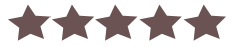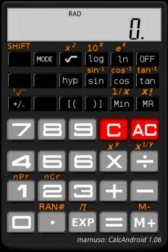Name - Scientific Calculator
Category - productivity
Resolution - multi
Type - Android 1.6 Donut
Size - 238 KB
Rating - 0/5 from 8 ratings
Description:
Ver 1.19, for android 1.6 and up

Do you remember these nice old days?
CalcAndroid - Scientific calculator.
Looks, works and feels like the old ones.
Features:
* 10 digits display + 2 digit exponent ( range from -9.9e99 to 9.9e99 )
* Typical operations ( add, subtract, multiply, divide ), with virtually infinite brackets
* Power functions ( n-power, n-root, square, square root, cubic root )
* Logarithmic functions ( log10, ln, 10 power, exp )
* Single value memory
* Trigonometric functions ( sin, cos, tan, including inverse and hyperbolic )
* Three angle modes ( deg, rad, gra )
* Random number generator
* PI constant
* Permutation (nPr) and combinatorial number (nCr)